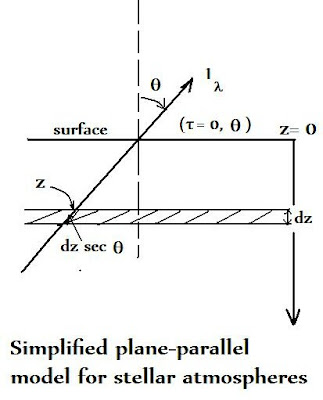## Wednesday, July 17, 2019

### Selected Questions- Answers From All Experts Astronomy Forum (Local Thermodynamic Equilibrium)

Question:  Can you clarify the meaning of 'local thermodynamic equilibrium' in relation to black body radiation for a star?  (P.S.  I am a math whiz!) - Margith, Salt Lake, UT

Answer:  It will be of use here to refer to the diagram below, which depicts the transfer of radiation for a star treated as having a "plane parallel" atmosphere, i.e. the stellar layers are essentially parallel;Of importance here is The Planck function describes the distribution of radiation for a black body, and can be expressed:

B(l) = {(2 hc2)/ l5}  [1/ exp (hc/lkT) - 1)]

Of course, all stars are effectively black bodies, which are perfect radiators, or as close to that state as nature allows.  In the case of simple radiation transfer in a static model stellar atmosphere (e.g. nothing changes with time), we have the relation of specific radiation intensity I(l) to source function S(l) at some specific wavelength,  l :

dI(l)/ds = -k(l)  I(l) + k(l)  S(l) =   k(l) [S(l) – I(l)] - 0   or I(l) = S(l

For a black body,   I(l) equals the Planck function:

i.e.  S(l) = I(l) = B(l

This is the condition which implies local thermodynamic equilibrium, or LTE.

LTE does NOT mean complete thermodynamic equilibrium,  since in the outer layers of a star there is always large energy loss from the stellar surface . Thus, one only assumes the emission of the radiation is the same as for a gas in thermodynamic equilibrium at a temperature (T) corresponding to the temperature of the layer under consideration.  Another way to say this is that if LTE holds, the photons always emerge at all wavelengths.  In the above treatment, note that the absorption coefficient was always written as: k(l) to emphasize its wavelength (l) dependence.

To make analysis even easier, one often uses the gray atmosphere approximation. In a particular instance, let the surface flux:

p( Fo ) = 2 p (I(cos (q)) = p [a(l) + 2(b(l)/3 ]

and:  Flo = S(l) t(l) = 2/3

This states that the flux coming out  of the  stellar surface is equal to the source function at the optical depth t = 2/3. This is the very important ‘Eddington-Barbier’ relation that facilitates an understanding of how stellar spectra are formed.

Once one then assumes LTE, one can further assume k(l) is independent of l (gray atmosphere) so that:

k(l) = kt (l) = t  and Flo =  Bl (T(t = 2/3) )

Thus, the energy distribution of Fl is that of a black body corresponding to the temperature at an optical depth t = 2/3.  From this, along with some simple substitutions and integrations a wide array of problems can be done.  Among the more interesting applications - since you are a math whiz - is to find the "equivalent width",  e.g. of a spectral line:

W =   ò ¥0  (I c – I u /  I c )duò ¥0  (F c – F l / F c ) dl

The left side defines W in terms of the intensity from the continuous spectrum outside the spectral line where the quantity (I c – I u / I c ) is referred to as the “depth of the line”, the analogous quantity to (F c – F l / F c ) on the right side where we have radiant flux units. Technically, the integral should be taken only from one side of the line to the other but the limits can be as shown provided I c (or  F c)  is kept constant in the neighborhood of the line.